Classical Mechanics

# Rotational Kinetic Energy - Problem Solving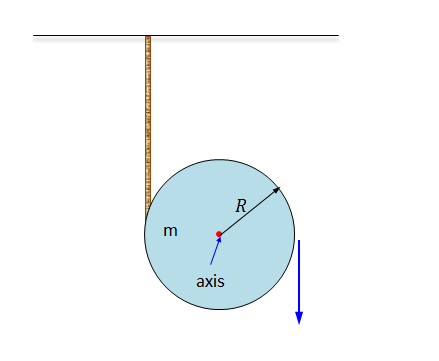A disk of mass $$m = 2 \text{ kg}$$ and radius $$R = 4 \text{ m},$$ which is wound with a rope hanging from the ceiling, starts to roll down. The linear velocity of the ball when it has rolled $$10 \text{ m}$$ down can be expressed as $$v = \sqrt{\frac{a}{b}} \text{ m/s},$$ where $$a$$ and $$b$$ are coprime positive integers. Find the value of $$a+b.$$

The gravitational acceleration is $$g=10\text{ m/s}^2.$$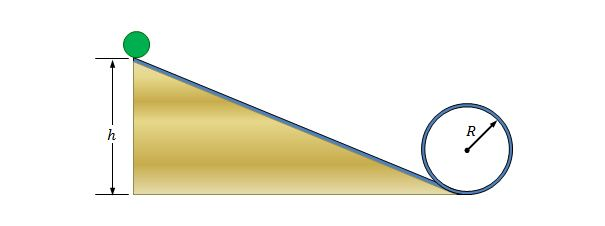A solid ball with radius $$r = 8 \text{ m}$$ initially at rest rolls down a down-hill track, and then proceeds through a circular loop track of radius $$R=30\text{ m}.$$ When the ball is at the highest point of the loop, it is traveling at the minimum speed required to stay on the track and not fall down. What was the initial height $$h$$ of the ball?

The gravitational acceleration is $$g=10\text{ m/s}^2.$$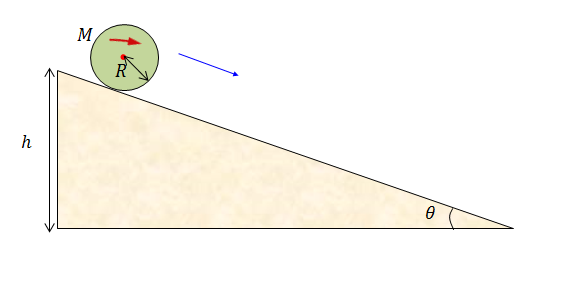A disk of mass $$M = 2 \text{ kg}$$ and radius $$R = 5 \text{ m}$$ starts to roll (without slipping) down an inclined plane that makes a $$\theta=30^\circ$$ angle with the horizontal. $$7$$ seconds later, the disk reaches the end of the inclined plane. If the disk's initial height can be expressed as $$h = {\frac{a}{b}} \text{ m},$$ where $$a$$ and $$b$$ are coprime positive integers, what is the value of $$a+b?$$

The gravitational acceleration is $$g=10\text{ m/s}^2.$$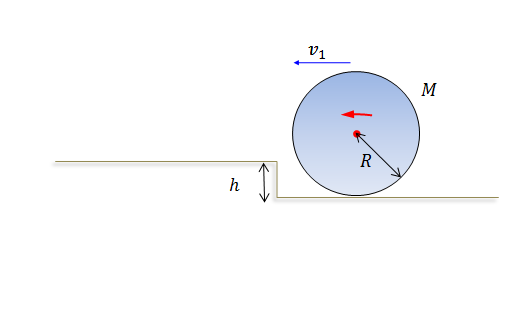A solid ball of mass $$m = 2 \text{ kg}$$ and radius $$R = 11 \text{ m}$$ is rolling on the ground with a linear velocity of $$v_1 = 11 \text{ m/s}.$$ Then the ball moves onto an elevated plane of height $$h = 1 \text{ m},$$ and its linear velocity can be expressed as $$v_2 = \sqrt{\frac{a}{b}} \text{ m/s},$$ where $$a$$ and $$b$$ are coprime positive integers. Find the value of $$a+b.$$

The gravitational acceleration is $$g=10\text{ m/s}^2.$$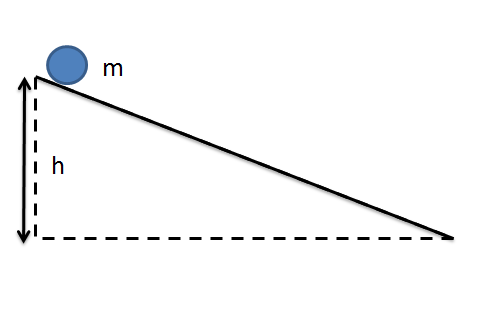A solid ball of mass $$m = 2 \text{ kg}$$ and radius $$r = 5 \text{ m}$$ initially at rest rolls down a hill whose height is $$h = 28 \text{ m}.$$ What is the ball's speed squared when it reaches the ground?

The gravitational acceleration is $$g=10\text{ m/s}^2.$$

×